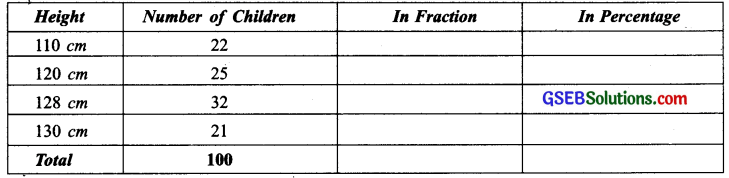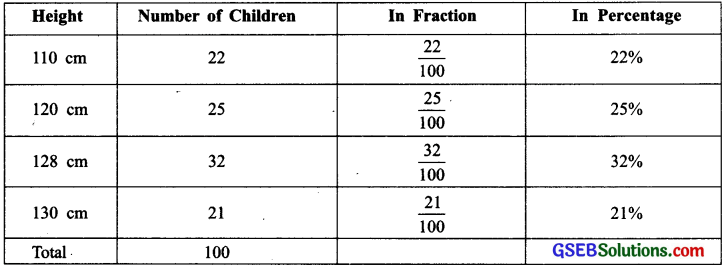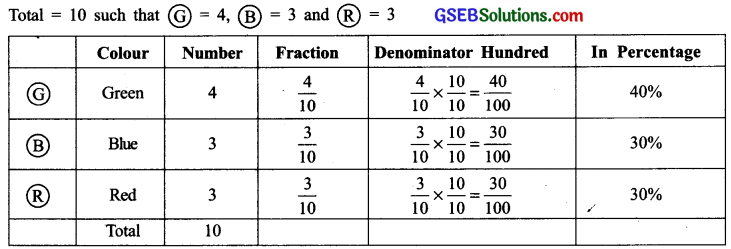# GSEB Solutions Class 7 Maths Chapter 8 Comparing Quantities InText Questions

Gujarat Board GSEB Textbook Solutions Class 7 Maths Chapter 8 Comparing Quantities InText Questions and Answers.

## Gujarat Board Textbook Solutions Class 7 Maths Chapter 8 Comparing Quantities InText QuestionsThink, Discuss and Write (Page 157)

Question 1.
An ant can carry 50 times its weight. If a person can do the same, how much would you be able to carry?
Solution:
I would be able to carry 50 times my weight.

Try These (Page 158)

Question 1.
Find the percentage of children of different heights for the following data.Solution:Question 2.
A shop has the following number of shoe pairs of different sizes.
Size 2 : 20 Size 3 : 30 Size 4 : 28 Size 5 : 14 Size 6 : 8
Write this information in tabular form as done earlier and find the percentage of each shoe size available in the shop.
Solution:Try These (Page 159)

Question 1.
A collection of 10 chips with different colours is given.Fill the table and find the percentage of chips of each colour.
Solution:
We haveQuestion 2.
Mala has a collection of bangles. She has 20 gold bangles and 10 silver bangles. What is the percentage of bangles of each type? Can you put it in the tabular form as done in the above example?
Solution:
We haveNote: We can use percentages for comparing various quantities.Think, Discuss and Write (Page 160)

Question 1.
Look at the examples below and in each of them, discuss which is better for comparison. In the atmosphere, 1 g of air contains:
(i) .78 g Nitrogen
.21% Oxygen
.01 g Other gas
or
78% Nitrogen
21 g Oxygen
1% Other Gas

(ii) A shirt has:
$$\frac { 3 }{ 5 }$$ Cotton
$$\frac { 2 }{ 5 }$$ Polyster
or
60% Cotton
40% Polyster
Solution:
The second one (i.e. percentage) is better for comparison in both the cases.Think, Discuss and Write (Page 161)

Question 1.
(i) Can you eat 50% of a cake l Can you eat 100% of a cake l Can you eat 150% of a cake?
(ii) Can a price of an item go up by 50%? Can a price of an item go up by 100%? Can a price of an item go up by 150%?
Solution:
(i) Yes, we can eat 50% of a cake.
Yes, we can eat 100% of a cake.
No, we cannot eat 150% of a cake.

(ii) Yes, a price of an item can go up by 50%. Yes a price of an item can go up by 100%. Yes, a price of an item can go up by 150%.

Try These (Page 161)

Question 1.
Convert the following to per cents:
(a) $$\frac { 12 }{ 16 }$$
(b) 3.5
(c) $$\frac { 49 }{ 50 }$$
(d) $$\frac { 2 }{ 2 }$$
(e) 0.05
Solution:Question 2.
(i) Out of 32 students, 8 are absent. What per cent of the students are absent?
(ii) There are 25 radios, 16 of them are out of order. What per cent of radios are out of order?
(iii) A shop has 500 parts, out of which 5 are defective. What per cent are defective?
(iv) There are 120 voters, 90 of them voted yes. What per cent voted yes?
Solution:
(i)∵$$\frac { 8 }{ 32 }$$ = $$\frac { 8 }{ 32 }$$ x 100% = 25%
∴ 25% students are absent.

(ii) ∵ $$\frac { 16 }{ 25 }$$ = $$\frac { 16 }{ 25 }$$ x 100%
= (16 x 4)% = 64%
∴ 64% radios are out of order.

(iii) ∵ $$\frac { 5 }{ 500 }$$ = $$\frac { 5 }{ 500 }$$ x 100% = 1%
∴ 1% parts are defective.

(iv) ∵ $$\frac { 90 }{ 120 }$$ x 100%
= (3 x 25)% = 75%
∴ 75% voters voted ‘Yes’.

Try These (Page 162)

Question 1.
Fill in the blanks:
(i) 35% + ______ % = 100%
(ii) 64% + 20% + ______ % = 100%
(iii) 45% = 100% – ______ %
(iv) 70% = ______ % – 30%
Solution:
(i) ∵ 100 – 35 = 65
∴ 35% + 65% = 100%

(ii) ∵ 64 + 20 = 84
and 100 – 84 = 16
∴ 64% + 20% + 16% = 100%

(iii) ∵ 100 – 45 = 55
∴ 45% = 100% – 55%

(iv) ∵ 70 + 30 = 100
∴ 70% = 100% – 30%

Question 2.
If 65% of students in a class have a bicycle, what per cent of the students do not have bicycles1
Solution:
Part of students having bicycles = 65%
∴ Remaining part of students = 100% – 65% = (100 – 65)% = 35%
Thus, 35% of students do not have bicycles.Question 3.
We have a basket full of apples, oranges and mangoes. If 50% are apples, 30% are oranges, then what per cent are mangoes?
Solution:
Quantity of apples = 50%
Quantity of oranges = 30%
∴ Quantity of mangoes in the basket = 100% – (50% + 30%)
= [100 – (50 + 30)]%
= [100 – 80]% = 20%.
Thus, the per cent of mangoes in the basket = 20%.

Think, Discuss and Write (Page 162)

Question 1.
Consider the expenditure made on a dress 20% on embroidery, 50% on cloth, 30% on stitching. Can you think of more such examples. Solution:
Do it yourself.

Try These (Page 163)

Question 1.
What per cent of these figures are shaded?Solution:
(i) Number of parts of the figure = 4
∴ Each part is $$\frac { 1 }{ 4 }$$ of the whole figure.
Number of parts shaded = 3
∴ Percentage of the shaded parts = $$\frac {3 }{ 4 }$$ x 100%
= (3 x 25)%
= 75%

(ii) Shaded parts of the tangram are: $$\frac { 1 }{ 4 }$$, $$\frac { 1 }{ 8 }$$ and $$\frac { 1 }{ 8 }$$
∴ Total shaded parts = $$\frac { 1 }{ 4 }$$ + $$\frac { 1 }{ 8 }$$ + $$\frac { 1 }{ 8 }$$ = $$\frac { 2+1+1 }{ 8 }$$
= $$\frac { 4 }{ 8 }$$
= $$\frac { 1 }{ 2 }$$
∴ Percentage of the shaded parts = $$\frac { 1 }{ 2 }$$ x 100% = 50%Try These (Page 164)

Question 1.
Find:
(a) 50% of 164
(b) 75% of 12
(c) 12$$\frac { 1 }{ 2 }$$% of 64
Solution:
(a) 50% of 164
∵ 50% = $$\frac { 50 }{ 100 }$$
∴ 50% of 164 = $$\frac { 50 }{ 100 }$$ of 164
= $$\frac { 50 }{ 100 }$$ x 164= 1 x 82 = 82
Thus, 50% of 164 = 82

(b) 75% of 12
75% of 12 = $$\frac { 75 }{ 100 }$$ of 12
= $$\frac { 75×12 }{ 100 }$$
= 3 x 3 = 9
Thus, 75% of 12 = 9

(c) 12$$\frac { 1 }{ 2 }$$% of 64
12$$\frac { 1 }{ 2 }$$% of 64 = ( $$\frac { 25 }{ 2 }$$ x $$\frac { 1 }{ 100 }$$ ) of 64
= $$\frac { 25 }{ 2 }$$ x $$\frac { 1 }{ 100 }$$ x 64 = 8
Thus, 12$$\frac { 1 }{ 2 }$$% of 64 = 8.

Question 2.
8% children of a class of 25 like getting wet in the rain. How many children like getting wet in the rain?
Solution:
8% of 25 = $$\frac { 8 }{ 100 }$$ of 25
= $$\frac { 8 }{ 100 }$$ x 25
= $$\frac { 8 }{ 4 }$$ = 2
∴ 2 children like getting wet in rain.Try These (Page 164)

Question 1.
9 is 25% of what number?
Solution:
Let the required number be x
∴ 25% of x = 9
or $$\frac { 25 }{ 100 }$$ × x = 9 ⇒ x = $$\frac { 9×100 }{ 25 }$$
or x = 9 x 4 = 36
Thus, 9 is 25% of 36.

Question 2.
75% of what number is 15?
Solution:
Let the required number be x
∴ 75% of x = 15
or $$\frac { 75 }{ 100 }$$ of x = 15
or $$\frac { 75 }{ 100 }$$ × x = 15
⇒ x = $$\frac { 15×100 }{ 75 }$$ = 20
Thus, 75% of 20 is 15.

Try These (Page 166)

Question 1.
Divide 15 sweets between Manu and Sonu so that they get 20% and 80% of them respectively.
Solution:
Share of Manu = 20% of 15 sweets
= $$\frac { 20 }{ 100 }$$ x 15 sweets
= 3 sweets
Share of Sonu = 80% of 15 sweets 80
= $$\frac { 80 }{ 100 }$$ x 15
= 4 x 3 sweets
= 12 sweets.

Question 2.
If angles of a triangle are in the ratio 2:3:4. Find the value of each angle.
Solution:
We know that sum of three angles of a triangle = 180°.
∵ The angles are in the ratio 2:3:4.
∴ Sum of the ratios = 2 + 3 + 4 = 9
∴ Angles are: $$\frac { 2 }{ 9 }$$ x 180°, $$\frac { 3 }{ 9 }$$ x 180° and $$\frac { 4 }{ 9 }$$ x 180°
or (2 x 20)°, (3 x 20)° or 40°, 60° and 80°.Try These (Page 167)

Question 1.
Find percentage of increase or decrease:
(i) Price of shirt decreased from ₹ 80 to ₹ 60.
(ii) Marks in a test increased from 20 to 30.
Solution:
(i) Initial price of the shirt = ₹ 80
Decreased price of the shirt = ₹ 60
Decrease in the price = ₹ 80 – ₹ 60 = ₹ 20
∴ Per cent decrease of price
= $$\frac { 20 }{ 80 }$$ x 100% = 25%

(ii) Initial marks = 20
Increased marks = 30
Increase in the marks = 30 – 20 = 10
∴ Per cent increase of marks = $$\frac { 10 }{ 100 }$$ x 100% = 50%

Question 2.
My mother says, in her childhood petrol was ₹ 1 a litre. It is ₹ 52 per litre today. By what percentage has the price gone up?
Solution:
Initial price = ₹ 1
Increased price = ₹ 52
∴ Increase in price = ₹ 52 – 1 = ₹ 51
Per cent increase = $$\frac { 51 }{ 1 }$$ x 100% = 5100%

Try These (Page 169)

Question 1.
A shopkeeper bought a chair for ₹ 375 and sold it for ₹ 400. Find the gain percentage.
Solution:
We have
CP of the chair = ₹ 375
SP of the chair = ₹ 400
∵ CP < SP, so there is a profit.
∴ Profit (or gain) = SP – CP
= ₹ 400 – ₹ 375 = ₹ 25
∴ Profit % = $$\frac { ₹ 25 }{ ₹ 375 }$$ x 100%
= $$\frac { 5 }{ 75 }$$ x 100%
= $$\frac { 1 }{ 15 }$$ x 100%
= $$\frac { 20 }{ 3 }$$%
= 6$$\frac { 2 }{ 3 }$$%

Question 2.
Cost of an item is ₹ 50. It was sold with a profit of 12%. Find the selling price.
Solution:
We have
CP of the item = ₹ 50
Profit % = 12%
∴ Profit = 12% of ₹ 50
= ₹ $$\frac { 12 }{ 100 }$$ x 50= ₹ 6
∴ SP = CP + Profit
= ₹ 50 + ₹ 6 = ₹ 56Question 3.
An article was sold for ₹ 250 with a profit of 5%. What was its cost price?
Solution:
Let the cost price be ₹ x.
Profit % = 5%
∴ Profit = 5% of x = ₹ $$\frac { 5 }{ 100 }$$ × x = ₹ $$\frac { x }{ 20 }$$
∴ SP = CP + Profit
∴ ₹ 250 = ₹ x + $$\frac { x }{ 20 }$$ [∵ ₹ = ₹ 250]
or ₹ 250 = ₹$$\frac { 21 }{ 20 }$$x
0r x = $$\frac { 250×20 }{ 21 }$$
= $$\frac { 5000 }{ 21 }$$
= 238$$\frac { 2 }{ 21 }$$
Thus, cost price of the article = ₹238$$\frac { 2 }{ 21 }$$

Question 4.
An item was sold for ₹ 540 at a loss of 5%. What was its cost price?
Solution:
Let the cost price be ₹ x.
∵ Loss % = 5%
∴ Loss = 5% of ₹ x = ₹ $$\frac { 5 }{ 100 }$$ × x = ₹$$\frac { x }{ 20 }$$
Now SP = CP – Loss
= ₹(x – $$\frac { x }{ 20 }$$)
= ₹$$\frac { 19 }{ 20 }$$x
But SP = ₹ 540
∴ $$\frac { 19 }{ 20 }$$x = 540 ⇒ x = $$\frac { 540×20 }{ 19 }$$
= $$\frac { 10800 }{ 19 }$$
= 568$$\frac { 8 }{ 19 }$$
∴ The CP of the item is ₹ 568$$\frac { 8 }{ 19 }$$

Try These (Page 170)

Question 1.
₹ 10,000 is invested at 5% interest rate p.a. Find the interest at the end of one year.
Solution:
Principal (P) = ₹ 10,000
Rate (R) = 5% p.a.,
Time (T) = 1 year
∴ Simple interest = $$\frac { P×R×T }{ 100 }$$
= ₹$$\frac { 10,000×5×1 }{ 100 }$$
= ₹ 500.Question 2.
₹ 3 ,500 is given at 7% p.a. rate of interest. Find the interest which will be received at the end of two years.
Solution:
Principal (P) = ₹ 3,500
Rate (R) = 7% p.a.
Time (T) = 2 years
∴ Simple interest = $$\frac { P×R×T }{ 100 }$$
= ₹$$\frac { 3,500×7×2 }{ 100 }$$
= ₹ 35 x 7 x 2
= ₹ 490.

Question 3.
₹ 6,050 is borrowed at 6.5% rate of interest p.a. Find the interest and the amount to be paid at the end of 3 years.
Solution:
∴ Principal (P) = ₹ 6,050
Rate (R) = 6.5% p.a.
Time (T) = 3 years
∴ Simple interest = $$\frac { P×R×T }{ 100 }$$
= ₹$$\frac { 6,050×6.5×3 }{ 100 }$$
= ₹$$\frac { 6,050×65×3 }{ 1,000 }$$
= $$\frac { 605×65×3 }{ 100 }$$
= ₹ $$\frac { 117975 }{ 100 }$$
= ₹ 1179.75
Since, Amount = Principal + Interest
= ₹ 6,050 + ₹ 1,179.75 = ₹ 7,229.75
Thus, the amount to be paid at the end of 3 years = ₹ 7,229.75.Question 4.
₹ 7 ,000 is borrowed at 3.5% rate of interest p.a. borrowed for 2 years. Find the amount to be paid at the end of the second year.
Solution:
Principal (P) = ₹ 7,000
Rate (R) = 3.5% p.a.
Time (T) = 2 years
∴ Simple interest = $$\frac { P×R×T }{ 100 }$$
= ₹$$\frac { 7,000×3.5×2 }{ 100 }$$
= ₹ 70 x $$\frac { 35 }{ 10 }$$ x 2
= ₹ 7 x 35 x 2
= ₹ 490
Since, Amount = Principal + Interest
= ₹ 6,050 + ₹ 1,179.75 = ₹ 7,229.75
Since, Amount = Principal + Interest
∴ Amount to be paid at the end of 2nd year = ₹ 7,000 + ₹ 490 = ₹ 7,490.

Try These (Page 171)

Question 1.
You have ₹ 2,400 in your account and the interest rate is 5%. After how many years would you earn ₹ 240 as interest.
Solution:
Here, principal (P) = ₹ 2,400
Rate (R) = 5% p.a.
Time (T) = ?
Simple interest = ₹ 240
∵ Simple interest = $$\frac { P×R×T }{ 100 }$$
∴ 240 = $$\frac { 240×5×T }{ 100 }$$
∴ T = $$\frac { 240×100 }{ 2400×5 }$$ = 2 years
Thus, an interest of ₹ 240 will be obtained after 2 years.Question 2.
On a certain sum the interest paid after 3 years is ₹ 450 at 5% rate of interest per annum. Find the sum.
Solution:
Principal (P) = ?
Rate (R) = 5% p.a.
Time (T) = 3 years.
Interest = ₹ 450
∵ Interest = $$\frac { P×R×T }{ 100 }$$
∴ 450 = $$\frac { P×5×3 }{ 100 }$$
or p = $$\frac { 450×100 }{ 5×3 }$$
= 30 x 100 = ₹ 3000
∴ The required sum (Principal) is ₹ 3000